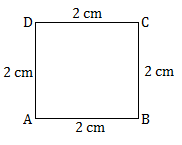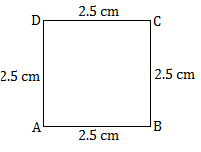# Perimeter of square Notes - Class 7

## Class 7: Perimeter of square Notes - Class 7

The document Perimeter of square Notes - Class 7 is a part of Class 7 category.
All you need of Class 7 at this link: Class 7

Perimeter of square?
Ref: https://edurev.in/question/438850/Perimeter-of-square-

Perimeter of a squareis the total length (distance) of the boundary of a square.

We know that all the sides of a square are equal.Perimeter of the square ABCD

= AB + BC + CD + AD

= 2 cm + 2 cm + 2 cm + 2 cm

= (2 × 4) cm

= 8 cm

Perimeter of a square is 4 times of s side.

Perimeter of a square = 4 × length of a side.

Examples on perimeter of a square:

1.Each side of a square is 2.5 cm. Find its perimeter.

Side = 2.5 cm

Therefore, perimeter of a square = 4 × length of a side

= (4 × 2.5) cm

2. Find the perimeter of a square shaped playground whose each side length 12 m.

We know a square has four equal sides. So, we can easily calculate the perimeter of a square.

The formula for finding the perimeter of a square is:

P = 4 × length of a side

= 4 × 12 m

= 48 m

3. Each sides of a paper sheet is 14 ft. Find its perimeter.

Given: Side length is 14 ft.

Perimeter of a square is = 4 × side

= 4 × 14 ft

= 56 ft

= 10 cm

The document Perimeter of square Notes - Class 7 is a part of Class 7 category.
All you need of Class 7 at this link: Class 7Use Code STAYHOME200 and get INR 200 additional OFF

Track your progress, build streaks, highlight & save important lessons and more!

,

,

,

,

,

,

,

,

,

,

,

,

,

,

,

,

,

,

,

,

,

;